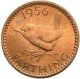## Removing duplicates using itertools.groupby

### Eliminating duplicates

Previously I discussed merging sorted streams using Python; a call to a standard library function for those who’ve updated to 2.6.

```>>> from heapq import merge
>>> from itertools import count, imap, islice
>>> m2, m3, m5 = [imap(n.__mul__, count(1)) for n in (2, 3, 5)]
>>> m235 = merge(m2, m3, m5)
>>> list(islice(m235, 10))
[2, 3, 4, 5, 6, 6, 8, 9, 10, 10]

```

Here, we merge positive multiples of 2, 3 and 5 into a single stream. 6 appears twice in the output, being a multiple of both 2 and 3. 10 is similarly duplicated and 30 would feature three times.

Itertools.groupby can remove the repeated entries.

```>>> from itertools import groupby
>>> help(groupby)
Help on class groupby in module itertools:

class groupby(__builtin__.object)
|  groupby(iterable[, keyfunc]) -> create an iterator which returns
|  (key, sub-iterator) grouped by each value of key(value).

```

If the key function is not specified or is `None` it defaults to an identity function, and `groupby` partitions an iterable into subiterators over equal valued elements.

```>>> from operator import itemgetter
>>> first = itemgetter(0)
>>> m235 = merge(*(imap(n.__mul__, count(1)) for n in (2, 3, 5)))
>>> u235 = imap(first, groupby(m235))
>>> list(islice(u235, 10))
[2, 3, 4, 5, 6, 8, 9, 10, 12, 14]

```

Groupby is very like the Unix uniq tool.

Generally (the documentation goes on to say) “the iterable needs to already be sorted on the same key function” but `groupby` is perfectly well defined when this isn’t the case. Here’s an artificial example showing groupby turning a random sequence into an alternating one.

### Random sequence

Let’s toss a coin to generate a random sequence of heads and tails.

```>>> def toss_a_coin():
...     import random
...     return random.choice("HT")
...
>>> from itertools import repeat
>>> def call(f):
...     return f()
...
>>> tosses = imap(call, repeat(toss_a_coin))
>>> spacer = ' '.join
>>> spacer(list(islice(tosses, 7)))
'T T H T H H H'

```### Alternating sequence

We can filter the unique values from this random sequence of coin tosses to generate an alternating sequence.

```>>> def uniq(seq):
...     return imap(first, groupby(seq))
...
>>> spacer(list(islice(uniq(tosses), 7)))
'H T H T H T H'

```### Constant sequence

Every other element of this filtered sequence will be the same.

```>>> tails = islice(uniq(tosses), 0, None, 2)
>>> spacer(list(islice(tails, 7)))
'T T T T T T T'

```Applying `uniq` again gives a single element infinitely repeated.

```>>> tt = uniq(tails)
>>> tt.next()
'T'
>>> tt.next()
C-c C-cTraceback (most recent call last):
File "<stdin>", line 1, in <module>
File "<stdin>", line 2, in call
File "<stdin>", line 2, in toss_a_coin
KeyboardInterrupt

```### Uniq -c

Imitating `uniq -c`, we can yield a repeat count paired to each unique value.

```>>> def ilen(it):
...     return sum(1 for _ in it)
...
>>> def counted_uniq(seq):
...     return ((ilen(i), k) for k, i in groupby(seq))
...
>>> ht = counted_uniq(tosses)
>>> list(islice(ht, 5))
[(1, 'H'), (3, 'T'), (1, 'H'), (3, 'T'), (3, 'H')]

```

How long before we see a run of 7 equal throws?

```>>> from itertools import takewhile
>>> tosses = imap(call, repeat(toss_a_coin))
>>> counts = imap(first, counted_uniq(tosses))
>>> sum(takewhile(lambda n: n < 7, counts))
44

```### Progression

The coin illustrating this note is a 1956 farthing, a small coin with a value of just ¼ of a penny dating from a time when there were 240 pence in every pound. The reverse features a wren, the obverse Queen Elizabeth II. The farthing was demonetised in 1960. In 1971 British currency went decimal and now, 37 years later, the 1971 coin designs are being revamped. Hiding amongst the loose change in my pocket today I noticed a couple of shiny new 2008 pennies. It’s about time we got euros.